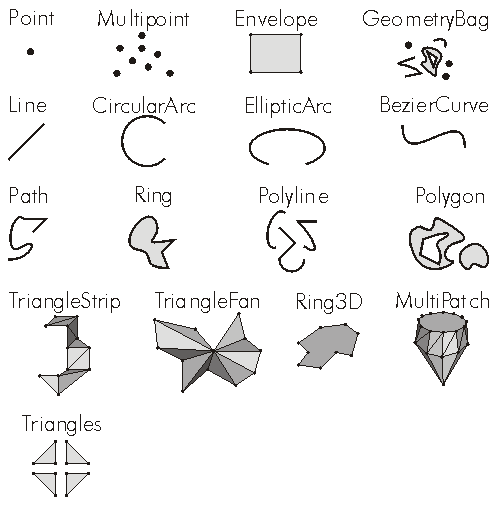This document is archived and information here might be outdated.  Recommended version.

IGeometry.GeometryType Property (ArcObjects .NET 10.7 SDK)
 ArcObjects Help for .NET developers > ArcObjects Help for .NET developers > ArcObjects namespaces > Geometry > ESRI.ArcGIS.Geometry > Interfaces > IG > IGeometry Interface > IGeometry.GeometryType Property
 ArcGIS Developer Help

# IGeometry.GeometryType Property

The type of this geometry.

```[Visual Basic .NET]
Public ReadOnly Property GeometryType As esriGeometryType
```
```[C#]
public esriGeometryType GeometryType {get;}
```
```[C++]
HRESULT get_GeometryType(
esriGeometryType* shapeType
);
```
```[C++]
Parameters
shapeType [out, retval]
shapeType is a parameter of type esriGeometryType*
```

#### Product Availability

Available with ArcGIS Engine, ArcGIS Desktop, and ArcGIS Server.

#### Remarks

```esriGeometryNull          = 0esriGeometryPoint         = 1esriGeometryMultipoint    = 2esriGeometryPolyline      = 3esriGeometryPolygon       = 4esriGeometryEnvelope      = 5esriGeometryPath          = 6esriGeometryAny           = 7esriGeometryMultiPatch    = 9esriGeometryRing          = 11esriGeometryLine          = 13esriGeometryCircularArc   = 14esriGeometryBezier3Curve  = 15esriGeometryEllipticArc   = 16esriGeometryBag           = 17esriGeometryTriangleStrip = 18esriGeometryTriangleFan   = 19esriGeometryRay           = 20esriGeometrySphere        = 21
```